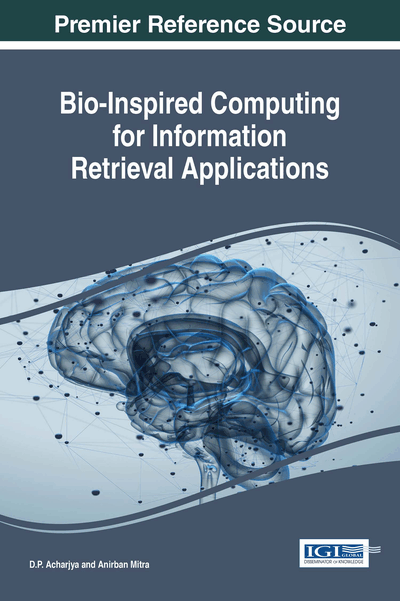# Generating Efficient Techniques for Information Extraction and Processing Using Cellular Automata

Subrata Paul (Vignan Institute of Technology and Management, India) and Anirban Mitra (Vignan Institute of Technology and Management, India)
DOI: 10.4018/978-1-5225-2375-8.ch008
Available
\$37.50
No Current Special Offers

## Abstract

The evolution of Cellular automaton has proved to be very efficient in carrying out arbitrary information processing. A significant application lies in the theory and practice of finding a technique for unifying the information processing. But, in this case the structures used in conventional computer languages are largely inappropriate. The definite organization of computer memory into named areas, stacks, and so on, is not suitable for cellular automata in which processing elements are not distinguished from memory elements. Rather it can be assumed that the data could be represented by an object like a graph, on which transformations can be performed in parallel. This chapter initiate with basic literature on cellular automata, related definitions and notations and focuses on its applications in information processing.
Chapter Preview
Top

## 1. Introduction

Automata theory is an exciting, theoretical branch of computer science. It established its roots during the 20th Century, as mathematicians began developing - both theoretically and literally - machines which imitated certain features of man, completing calculations more quickly and reliably. The word automaton itself, closely related to the word “automation”, denotes automatic processes carrying out the production of specific processes. Simply stated, it deals with the logic of computation with respect to simple machines, referred to as automata. Through automata, computer scientists are able to understand how machines compute functions and solve problems and more importantly, what it means for a function to be defined as computable or for a question to be described as decidable (Yan, 1998).

The major objective of automata theory is to develop methods by which computer scientists can describe and analyse the dynamic behaviour of discrete systems, in which signals are sampled periodically. The behaviour of these discrete systems is determined by the way that the system is constructed from storage and combinational elements (Chakraborty, Saxena & Katti, 2011). Characteristics of such machines include inputs, outputs and states. Inputs are assumed to be sequences of symbols selected from a finite set I of input signals. Namely, set I is the setwhereis the number of inputs. Outputs are sequences of symbols selected from a finite set Z. Namely; set Z is the setwherethe number of outputs is. States are denoted as Q, whose definition depends on the type of automaton.

There are four major families of automaton. These are finite state machine, pushdown automata, linear bounded automata, and Turing machine. The families of automata can be interpreted in a hierarchal form, where the finite state machine is the simplest automata and the Turing machine is the most complex. A Turing machine is a finite state machine yet the inverse is not true (Amadek & Trnkova, 1990). The following Figure 1 depicts the families of automaton.

Top

## 2. Finite Automata

A finite automaton is the mathematical model of some machine whose state may change in time, the set of possible states being finite. Its behaviour is the succession of its states throughout time. Its characteristically features are the set of states, Q, and the rules for their changes. The number of states being finite, there is no question of a continuous course, so the time-scale will be N. Changes are ruled by the function δ mapping state of automaton at time t, denoted, on state at timeThe behaviour of the automaton is studied from an initial state, it is the sequence:

As just described, isolated and independent from any outer surrounding, automaton presents very little interest; indeed, if k is the number of states in Q, two out of the (k + 1) states from time 0 to time k must be the same (Lane, 1971).such that## Complete Chapter List

Search this Book:
Reset